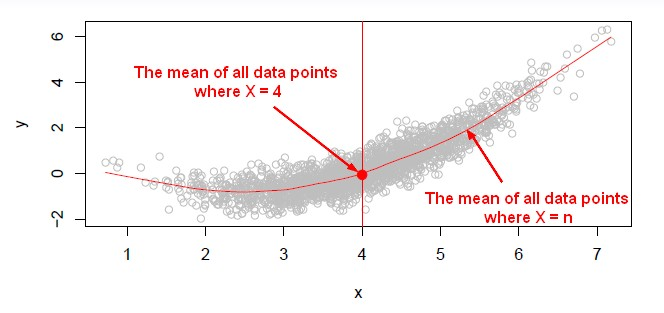# R - K-Nearest Neighbors (KNN) Analysis

## Steps

### Prerequisites

````library(class)`
```

### Syntax

``````?knn
knn(train, test, cl, k = 1, l = 0, prob = FALSE, use.all = TRUE)```
```

where:

• k is number of neighbours to be considered.
• train is the training set
• c1 is the factor of the training set with the true target
• test is the test set

### Training and Test Data set

• The knn function is waiting for two matrix (a training set and a test set)
``````# To be able to call all data frame variables by names
attach(myDataFrame)

#  Make a matrix of the chosen variables variable1 and variable1
variables=cbind(variable1,variable2)

# Make an indicator (a vector of true or false)
indicator=variableName<10

# The training set will be then
variables[indicator,]
# And the test set will be:
variables[!indicator,]```
```

### Model

Call to the knn function to made a model

````knnModel=knn(variables[indicator,],variables[!indicator,],target[indicator]],k=1)`
```

To classify a new observation, knn goes into the training set in the x space, the feature space, and looks for the training observation that's closest to your test point in Euclidean distance and classify it to this class.

### Accuracy

#### Confusion Matrix

````table(knnModel,variables[!indicator])`
```
``````knnModel  False True
False    43   58
True     68   83
```
```

#### Mean

````mean(knnModel==variables[!indicator])`
```
`````` 0.5
```
```

It was useless as One nearest neighbor did no better than flipping a coin.

### Next

We could proceed further and try nearest neighbors with multiple values of k.

Discover MoreMachine Learning - K-Nearest Neighbors (KNN) algorithm - Instance based learning

“Nearest‐neighbor” learning is also known as “Instance‐based” learning. K-Nearest Neighbors, or KNN, is a family of simple: classification and regression algorithms based on Similarity...